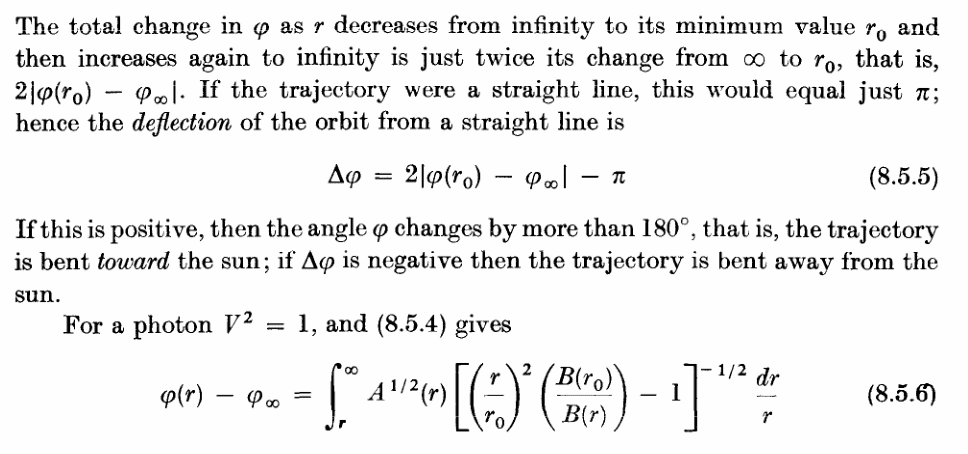# General form of the light deflection formula

• I
andresB
TL;DR Summary
looking for general formulas for the angle shift in terms of the impact parameter.
I know the basics of GR, but I'm far from an expert. I'm interested in the general formula for the angle shift experienced by a light ray in presence of a gravitational field. Light bending is topic covered in many books, of course, however the usual treatments would just not do for my purposes.

Most of the books I have looked at are just interested in the Schwarzschild metric, and take it from granted from the very beginning. The most general treatment I've found is in Weinberg chapter 8 where most of the treatment is metric agnostic and Schwarzschild is only assumed at the very end. However, the angle shift formula given by Weinberg is in terms of the "distance of closest approach", and that's not useful to me.

I'm looking for a general formula that is given in terms of the impact parameter.

Gold Member
Summary:: looking for general formulas for the angle shift in terms of the impact parameter.

I'm interested in the general formula for the angle shift experienced by a light ray in presence of a gravitational field.
And the one in the Wiki article isn't general enough?

andresB
And the one in the Wiki article isn't general enough?

Hi. Are you talking about the gravitational lens page? if that's the case then no. The page give the end result of the calculation for the Schwarzschild metric, and I need the formula for an arbitrary (spherically symmetric) metric.

To be more clear, this is the formula given in Weinberg.I need something like Eq (8.5.6) but in terms of the impact parameter ##b## and not the "distance of closest approach" ##r_{0}##. Or, better yet, assuming I already have ##\Delta\phi## in terms of ##r_{0}## how to change it to be a function of ##b##.

Mentor
I need the formula for an arbitrary (spherically symmetric) metric.

The Schwarzschild metric is the only spherically symmetric vacuum metric, by Birkhoff's theorem, so it's the only spherically symmetric metric you need to worry about if you're looking at light propagation through a vacuum.

If you're looking at light propagation through materials, any gravitational effects are going to be extremely tiny compared to refraction and other optical effects caused by the material.

Mentor
I need something like Eq (8.5.6) but in terms of the impact parameter ##b## and not the "distance of closest approach" ##r_{0}##.

##r_0## is the impact parameter in the weak field limit. I haven't looked at Weinberg in quite a while, but IIRC he is working in the weak field limit in the derivation you refer to.

The real issue is that ##r_0## is the minimum radial coordinate, which is not the same as a radial proper distance. But in the weak field limit, the difference is negligible.

Staff Emeritus
Homework Helper
Gold Member
If you're looking at light propagation through materials, any gravitational effects are going to be extremely tiny compared to refraction and other optical effects caused by the material.

There are a couple of cases of physical interest where the other optical effects are not overwhelming, such as lensing by an extended dark matter halo or a very weakly interacting massless particle moving through a spherical object. In these situations, it does make sense to think about deflection in a non-Schwarzschild spacetime.

Or, better yet, assuming I already have Δϕ in terms of r0 how to change it to be a function of b.

You can find ##b## in terms of ##r_0## as they are intimately related. If you know ##r_0## then you know the angular momentum and if you know the angular momentum you know ##b##.

andresB
There are a couple of cases of physical interest where the other optical effects are not overwhelming, such as lensing by an extended dark matter halo or a very weakly interacting massless particle moving through a spherical object. In these situations, it does make sense to think about deflection in a non-Schwarzschild spacetime.

Or situations in analogue gravity.

You can find ##b## in terms of ##r_0## as they are intimately related. If you know ##r_0## then you know the angular momentum and if you know the angular momentum you know ##b##.

Ok. I'll try to relate the two quantities, it might be the easiest path.

andresB
##r_0## is the impact parameter in the weak field limit. I haven't looked at Weinberg in quite a while, but IIRC he is working in the weak field limit in the derivation you refer to.

The real issue is that ##r_0## is the minimum radial coordinate, which is not the same as a radial proper distance. But in the weak field limit, the difference is negligible.

You are correct, for a Schwarzschild the two quantities are almost the same in a weak field situation. It might be the same for the metrics I'm considering, though I'll have to check.